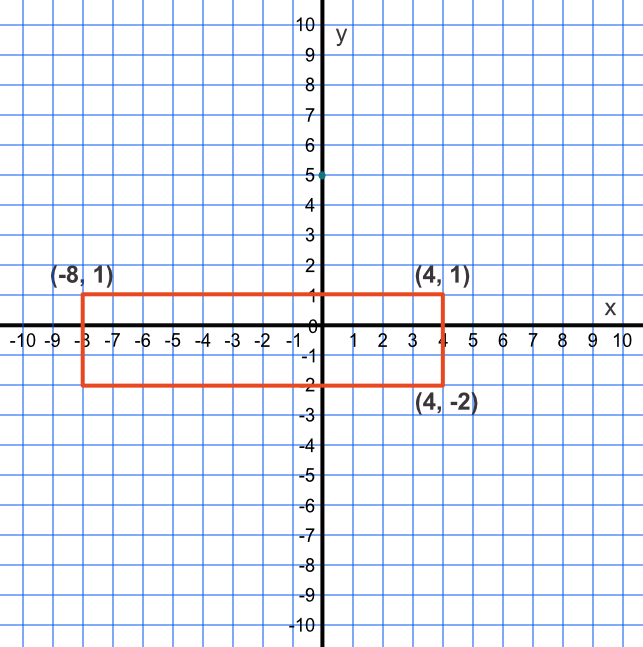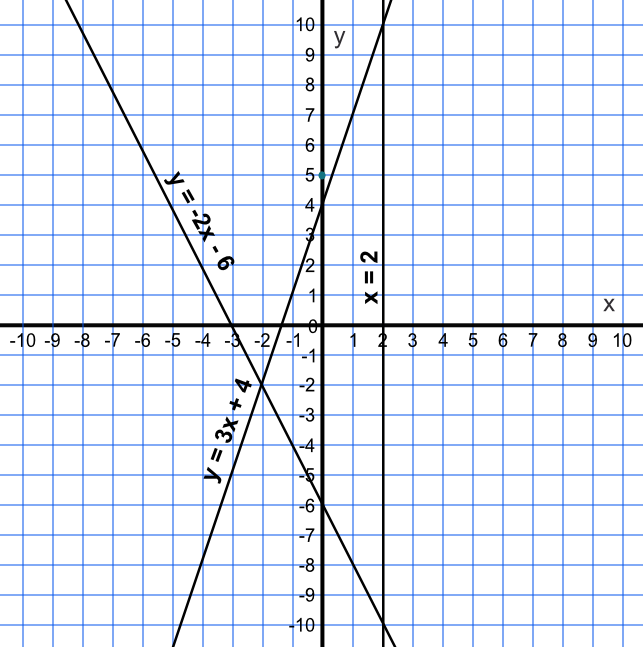Geometric Information on a Graph

## Geometric Information on a Graph

Graphs can be used to support geometric information: vertices (or corners) can be defined by coordinates, edges can be defined by equations or line segments, and the centre of a circle can be defined by a coordinate.

## Example 1

A rectangle has vertices at coordinates (-8,1), (4,1) and (4,-2).

What is the coordinate of the fourth vertex?

There must be two matching x-coordinates and two matching y-coordinates. The missing values are -8 for x and -2 for y. The missing coordinate is (-8,-2).## Example 2

A triangle is defined by three lines:

y=3x+4 , y = -2x -6 and x=2

What is the length between the highest and lowest points of the triangle?

Note that the line x=2 is vertical

For y=3x+4 when x=2 then y=10

The top coordinate is (2, 10)

For y = -2x-6 when x=2 then y=-10

The bottom coordinate is (2, -10)

Vertical distance is 10 - -10 = 20

If unsure, sketch the graph first